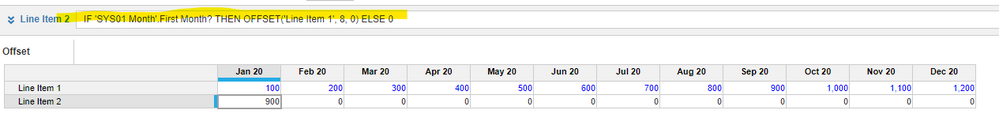# help with formulaHi,

I'm trying to create a function that divides two line items, but the second line item needs to be the value of the 8th month from the first line item. This is the function that I created.

'F01 Funding Breakdown'.Total Funding / IF MONTH(START()) = 1 THEN OFFSET('F01 Funding Breakdown'.Scaled New Originations, 8, 0) ELSE 0

I'm still not getting what I want with this function. please advise.

Thank you

Tagged:

•You have the best questions. Here's how I would approach this.

Instead of using the month(start()) equation which would have to be calculated at every cell, try using a system module that indicates which month is the first month.

You can set up a module that only uses the model calendar. Create a line item that is Boolean Formatted. Set the formula to find the first month. Call it "First Month". Then use that in your Line Item 2 formula, like this. The "8", by the way, can be a driver you set in another system module in case you need it to be more dynamic.•Wait Im kind of confused what the Boolean Line Item would be. Because I would want the formula to Divide the first Line Item by the Second Line Item, but the second line item needs to be the value eight months later.  So for example If it was February, I would need to divide by the value in October. If it was March, I would need to divide by November's value...and so forth

•Got it, I'm testing it out now. Thank you!